Technical Information Site of Power Supply Design

2018.05.24 Transfer Function

# Example of Derivation for a Step-Up/Step-Down Converter - 2

DC/DC Converters: Sharing of Transfer Functions among Control Systems

This section is the continuation of the previous “Example of Derivation for a Step-Up/Step-Down Converter – 1”. Various control methods are used in step-up/step-down converters. Here, we are focusing on two control methods among these, and in this section we consider the second of these.

As before, the transfer functions to be derived are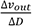and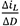, and once again, the transfer functions are derived in two steps.

Example 2 of Derivation of Transfer Functions for a Step-Up/Step-Down Converter: Step-Up/Step-Down Converter for which ton≠ton'

In the control method considered this time, the ON-times for the step-down switch and for the step-up switch are not the same, and the switches each operate independently. Hence depending on the relation between the input voltage and the output voltage, the behaviors of the step-down switch and the step-up switch vary, and there are transfer functions with a number of patterns. This time we shall derive the transfer functions for a region in which the step-down switch and the step-up switch are both switching.

To begin with, the specific control system is shown in Figure 13.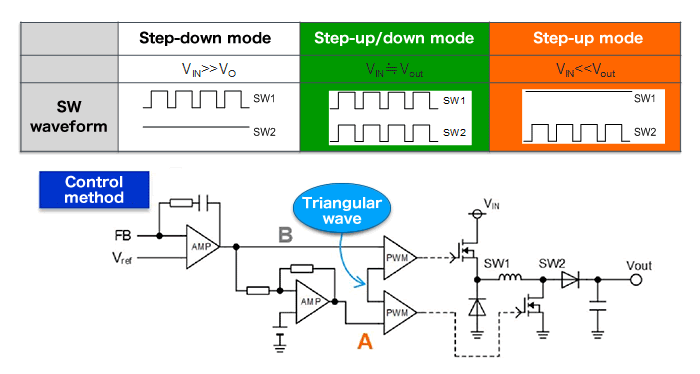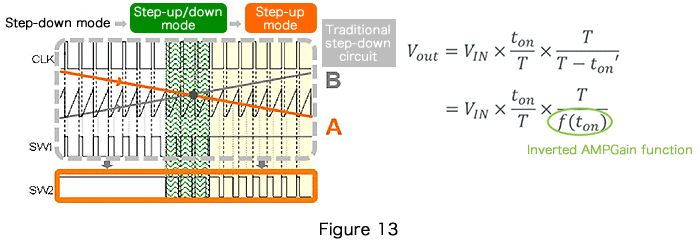Depending on the relation between input and output, there are three modes of operation--step-down, step-up/step-down, and step-up. The switching waveforms of SW1 and SW2 are shown. Their switching operation in each of these modes should be clear.

Step-Up/Step-Down Converters for which ton≠ton'

The circuit on the right is a simplified version of the above circuit. The equations are derived in two steps, as we have done before.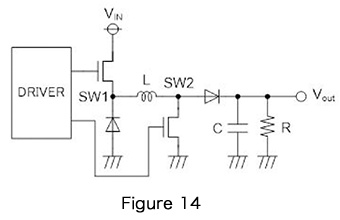●Step 1: Consider the stable states of the system

① The coil current does not change over one period
② The capacitor charge amount does not change
over one period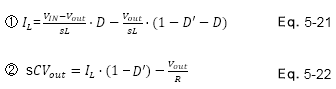●Step 2:
Determine change amounts for an external
disturbance, and describe the transfer functions

A calculation example from equations 5-21 and 5-22 above is shown below.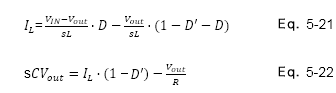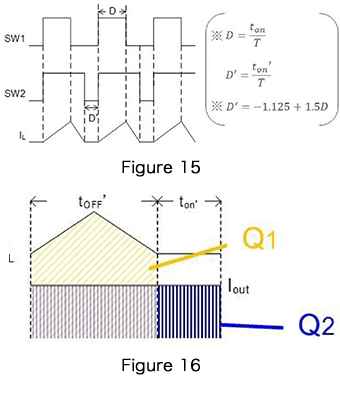Upon substituting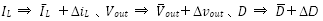in equations 5-21 and 5-22, the following is obtained.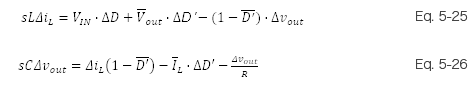Here, we set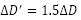.

Taking equations 5-25 and 5-26 as a system of simultaneous equations and determiningand, we obtain the following.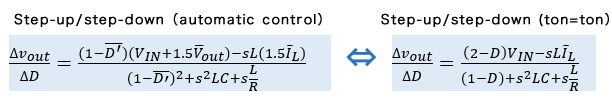As a result, the part indicating the duty is different, but similar results for characteristics are obtained.

Up to this point, we have derived transfer functions for step-down, step-up, and step-up/step-down converters, and we have used a unified approach in all cases. The reader should by all means try deriving the equations and doing the calculations. From the next time, we will consider these transfer functions with other factors included.

This website uses cookies.

By continuing to browse this website without changing your web-browser cookie settings, you are agreeing to our use of cookies.